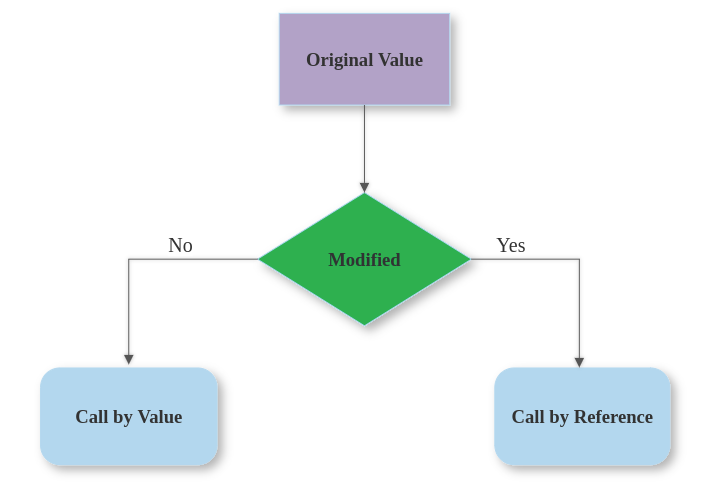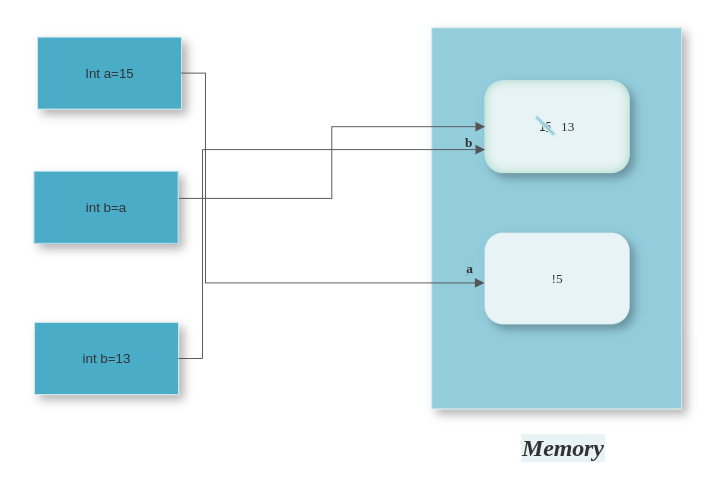In JavaScript, a variable may store two types of values, Primitive values or Reference values. This article will describe and help to compare both these types of values## Call by value

When a variable is passed by value, a copy of the variable’s value is passed to the function or method. Any changes made to the variable within the function or method do not affect the original variable outside of it. In JavaScript, primitive data types (such as numbers, strings, and booleans) are passed by value.

``````const addFunc=(x)=&gt;{
console.log( x +10);
}

let y=10;

console.log(y) //prints 10
``````

## Call by reference

When a variable is passed by reference, a reference to the memory location of the variable is passed to the function or method. Any changes made to the variable within the function or method are reflected in the original variable outside of it. In JavaScript, objects and arrays are passed by reference.#### Example

``````let num = 5;

let obj = {a: 1);

function changeValue(val) (
val = 10;

function change0bj(o) {
o.a = 2;
}
changeValue(num);

console. log(num); // still prints 5

change0bj(obj);

console. log(obj.a); // prints 2
``````

It’s important to keep in mind that when passing an object or array to a function and modifying it within the function, the original object or array is also modified. This can lead to unexpected behavior if not handled properly.

As a best practice, it’s a good idea to create a copy of an object or array before passing it to a function if you do not want the original to be modified.

#### Example

There are several ways to copy an object or array before passing it to a function.

• For Array
``````let originalArray = [1, 2, 3];

// copied Arrays
let copiedArray1 = originalArray.slice();

let copiedArray2 = [].concat(originalArray);

let copiedArray3 = [...originalArray];
``````
• For Object
``````let originalObj = {a: 1};

// copied Objects
let copiedObj1 = Object.assign({}, originalObj);

let copiedObj2 = {...originalObj};

let copiedObj3 = JSON.parse(JSON.stringify(originalObj));
``````

### Few Puzzles

##### Puzzle 1

What would be the value of a and b?

``````a = {z:10,x:20}

b =[{...a}]

b.z=20;

console.log(`a=\${a}`)

console.log(`b=\${b}`)
``````
``````a ={z : 10, x : 20} , b= [ { z : 20, x : 20 } ]
``````

Here the spread operator ( … ) copies the array. so change done to b is only effected in b.

##### Puzzle 2

What would be the result of the following consoles?

``````let a = [{z:10}];
let b = [...a];

// b=[{z:10}]

b.z =100;

// Part 1
console.log(`a=\${a}`)
console.log(`b=\${b}`)

//Part 2
b.push(40)
console.log(`a=\${a}`)
console.log(`b=\${b}`)

//Part 3
let c = [{...a}];
c.z=300;

console.log(`a=\${a}`)
console.log(`c=\${c}`)
``````
``````a= [ { z : 100 } ] , b= [ { z : 100 } ]
``````
``````a = [ { z : 100 } ] , b = [ { z :100 } ,40]
``````

In the code, an array a is created with an object {z: 10}. A shallow copy of a is created and assigned to b using the spread operator ( … ). When b.z is set to 100, the change is reflected in both a and b since it’s just a shallow copy. Adding an element to b (b.push(40)) only affects b and does not reflect in a.

`````` a = [ { z : 100 } ] , c = [ { z : 300 } ]